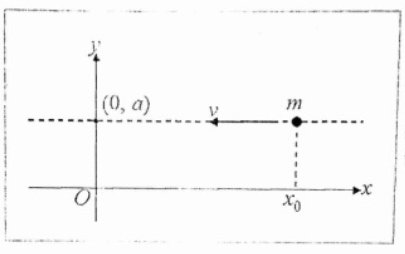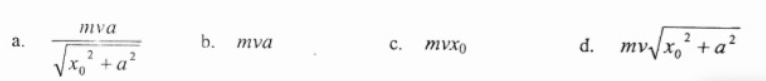# Problem: A particle of mass m moves with a constant speed v along the dashed line y = a. When the x-coordinate of the particle is x0, the magnitude of the angular momentum of the particle with respect to the origin of the system is

###### FREE Expert Solution

For the particle, the perpendicular distance between the velocity vector and the origin is a.

92% (483 ratings)###### Problem DetailsA particle of mass m moves with a constant speed v along the dashed line y = a. When the x-coordinate of the particle is x0, the magnitude of the angular momentum of the particle with respect to the origin of the system is